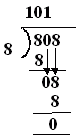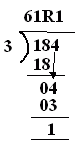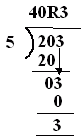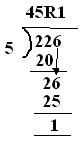Name: ___________________Date:___________________

 Email us to get an instant 20% discount on highly effective K-12 Math & English kwizNET Programs!

### Grade 3 - Mathematics10.1 Division - Dividend, Divisor, Quotient, Remainder

 Example:In the above division problem: Dividend is 808 Divisor is 8 Quotient is 101 Remainder is 0 Directions: Answer the following questions. Also write at least 10 examples of your own.Name: ___________________Date:___________________

### Grade 3 - Mathematics10.1 Division - Dividend, Divisor, Quotient, Remainder

 Q 1: In the division problem shown the divisor is ____.Answer: Q 2: In the division problem the quotient is ___.Answer: Q 3: In the division problem shown the dividend is ____.Answer: Q 4: In the division problem shown the divisor is ___.Answer: Q 5: In the division problem shown the remainder is ____.Answer: Q 6: In the division problem the remainder is ____.Answer: Question 7: This question is available to subscribers only! Question 8: This question is available to subscribers only!

#### Subscription to kwizNET Learning System costs less than \$1 per month & offers the following benefits:

• Unrestricted access to grade appropriate lessons, quizzes, & printable worksheets
• Instant scoring of online quizzes
• Progress tracking and award certificates to keep your student motivated
• Unlimited practice with auto-generated 'WIZ MATH' quizzes